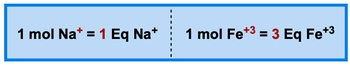Start typing, then use the up and down arrows to select an option from the list.# GOB Chemistry

Learn the toughest concepts covered in your GOB - General, Organic, and Biological Chemistry class with step-by-step video tutorials and practice problems.

Solutions

Equivalents are used to measure individual ion amount present in body fluids and intravenous solutions.

## Equivalents (Eq)

1

#### concept

Equivalents Concept 11m
Play a video:
equivalents are used to measure individual ion amount present in body fluids, an intravenous solutions here, we're going to say that an equivalent or abbreviated E Q. Is the number of moles of charge, That one I'll contributes to a solution. I want to say here that an equivalent equals one mole of positive or negative charge. One important thing to remember is that an equivalent can only be a positive value though. So for example, here we have one mole of sodium ion. The charge is Plus one. So we'd say we have one equivalent here, we say one mole of iron three I on the number of the charges, three, which equates to three equivalents of iron three ion. We'll see how to calculate that right below. We're gonna say to calculate the number of equivalent of an ion, we simply multiply ion charge for the number of moles of ion present. Here, we're going to say that a milli equivalent is a common unit used to express equivalents. And remember millie, so one equivalent equals 1000 milli equivalents. And when it comes to an equivalent, just remember, equivalent equals ion charge times the moles of ions. So if we went up above again, the charge here is one and the number of moles is one. So that's why I was one equivalent here. The mole, the ion charges three and it's just one mole of iron three ion. So that's why the equivalent was three. So just keep that in mind when asked to calculate the equivalent of any ionic solution.2

#### example

Equivalents Example 143s
Play a video:
calculate the number of equivalent in each of the following. Remember an equivalent equals the ion charge times the moles of ion. So for the first one, a ion charge calcium has a two plus charge. So the charges two times the moles of the ion, which is just one mole. That means we have two equivalents of calcium ion. For B, the ion charge of the phosphate ion is three minus, so that's three times the number of moles is two moles. That's six equivalents of the phosphate ion. So those will be your answers for both A and.
3

#### concept

Equivalents Concept 231s
Play a video:
Now that we've looked at equivalence, we can talk about normality. Non normality is the concentration of ions in Equus solution. And we're going to say that normality, which uses the variable capital N, represents the number of equivalence per liter of solution. Right? So just remember we've calculated equivalents before what's equal to the ion charge times the moles of ions. Now we're going to incorporate that understanding into this idea of normality, normality equals equivalents over leaders of solution.
4

#### example

Equivalents Example 21m
Play a video:
here we need to calculate the normality of 0.35 moles of magnesium ions present in 300 mL of blood. Alright, so normality equals your equivalence divided by your leaders of solution Here, we know what our leaders of solution are in a sense. We have 300 ml of blood. Blood. Is our solution here Converting that into leaders gives us .300 leaders. Now we need to figure out the number of equivalence. Remember your equivalence equals the ion charge times the moles of ions. So here the charge of magnesium is two plus. So that's two times 20.35 moles which is equal 2.7. So we have .7 equivalents. So when we do 7.7 divided by .3, that's gonna give us 2.33 Or just 3 normality. So this would be the normality of this particular solution.
5
Problem

Calculate mass (grams) needed for the following ion equivalent:1.5 mEq of Na+ ions.

6
Problem

The concentration of Cl- ion in blood is approximately 105 mEq/L. How many milliliters of blood would be needed to obtain 1.4 g of Cl- ions?

7
Problem

An intravenous saline solution contains 140 mEq/L of Na+. How many mEq of Na+ are present in 750 mL of the solution?

8
Problem

Calculate the normality (mEq/L) of potassium ions in a 500 mL Ringer’s solution that is 2.0 x 10-3 M in potassium ions.